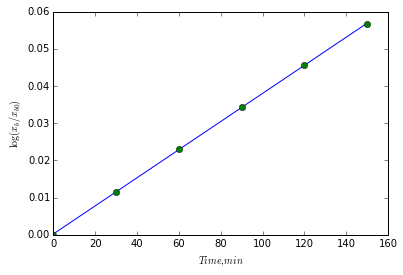# Chapter 17: Transport Phenomena¶

## Example Problem 17.1, Page Number 417¶

In :
from scipy import constants
from math import sqrt,pi

#Variable Declaration
M = 0.040                  #Molecualar wt of Argon, kh/mol
P, T = 101325.0, 298.0     #Pressure and Temperature, Pa, K
sigm = 3.6e-19  #
R = 8.314                  #Molar Gas constant,  mol^-1 K^-1
N_A = 6.02214129e+23       #mol^-1
#Calculations
DAr = (1./3)*sqrt(8*R*T/(pi*M))*(R*T/(P*N_A*sqrt(2)*sigm))

#Results
print 'Diffusion coefficient of Argon %3.1e m2/s'%DAr

Diffusion coefficient of Argon 1.1e-05 m2/s


## Example Problem 17.2, Page Number 418¶

In :
from math import sqrt

#Variable Declaration
DHebyAr = 4.0
MAr, MHe = 39.9, 4.0       #Molecualar wt of Argon and Neon, kg/mol
P, T = 101325.0, 298.0     #Pressure and Temperature, Pa, K
sigm = 3.6e-19  #
R = 8.314                  #Molar Gas constant,  mol^-1 K^-1
N_A = 6.02214129e+23       #mol^-1
#Calculations
sigHebyAr = (1./DHebyAr)*sqrt(MAr/MHe)

#Results
print 'Ratio of collision cross sections of Helium to Argon %4.3f'%sigHebyAr

Ratio of collision cross sections of Helium to Argon 0.790


## Example Problem 17.3, Page Number 420¶

In :
from math import sqrt

#Variable Declaration
D = 1.0e-5                 #Diffusion coefficient, m2/s
t1 = 1000                  #Time, s
t10 = 10000                #Time, s

#Calculations
xrms1 = sqrt(2*D*t1)
xrms10 = sqrt(2*D*t10)

#Results
print 'rms displacement at %4d and %4d is  %4.3f and %4.3f m respectively'%(t1,t10,xrms1,xrms10)

rms displacement at 1000 and 10000 is  0.141 and 0.447 m respectively


## Example Problem 17.4, Page Number 421¶

In :
#Variable Declaration
D = 2.2e-5                 #Diffusion coefficient of benzene, cm2/s
x0 = 0.3                   #molecular diameter of benzene, nm

#Calculations
t = (x0*1e-9)**2/(2*D*1e-4)

#Results
print 'Time per random walk is %4.3e s or %4.2f ps'%(t,t/1e-12)

Time per random walk is 2.045e-11 s or 20.45 ps


## Example Problem 17.5, Page Number 424¶

In :
from math import sqrt,pi

#Variable Declaration
P = 101325                  #Pressure, Pa
kt = 0.0177                 #Thermal conductivity, J/(K.m.s)
T = 300.0                   #Temperature, K
k = 1.3806488e-23           #Boltzmanconstant,J K^-1
sigm = 3.6e-19  #
R = 8.314                  #Molar Gas constant,  mol^-1 K^-1
NA = 6.02214129e+23        #mol^-1
M = 39.9                   #Molecualar wt of Argon and Neon, kg/mol

#Calculations
CvmbyNA = 3.*k/2
nuavg = sqrt(8*R*T/(pi*M*1e-3))
N = NA*P/(R*T)
labda = 3*kt/(CvmbyNA*nuavg*N)
sigm = 1/(sqrt(2)*N*labda)

#Results
print 'Mean free path %4.3e m and collisional cross section %4.2e m2'%(labda, sigm)

Mean free path 2.627e-07 m and collisional cross section 1.10e-19 m2


## Example Problem 17.6, Page Number 427¶

In :
from math import sqrt,pi

#Variable Declaration
eta = 227.                  #Viscosity of Ar, muP
P = 101325                  #Pressure, Pa
kt = 0.0177                 #Thermal conductivity, J/(K.m.s)
T = 300.0                   #Temperature, K
k = 1.3806488e-23           #Boltzmanconstant,J K^-1
R = 8.314                   #Molar Gas constant,  mol^-1 K^-1
NA = 6.02214129e+23         #mol^-1
M = 39.9                    #Molecualar wt of Argon and Neon, kg/mol

#Calculations
nuavg = sqrt(8*R*T/(pi*M*1e-3))
N = NA*P/(R*T)
m = M*1e-3/NA
labda = 3.*eta*1e-7/(nuavg*N*m)          #viscosity in kg m s units
sigm = 1./(sqrt(2)*N*labda)

#Results
print 'Collisional cross section %4.2e m2'%(sigm)

Collisional cross section 2.74e-19 m2


## Example Problem 17.7, Page Number 429¶

In :
from math import sqrt,pi

#Variable Declaration
m = 22.7                    #Mass of CO2, kg
T = 293.0                   #Temperature, K
L = 1.0                     #length of the tube, m
d = 0.75                    #Diameter of the tube, mm
eta = 146                   #Viscosity of CO2, muP
p1 = 1.05                   #Inlet pressure, atm
p2 = 1.00                   #Outlet pressure, atm
atm2pa = 101325             #Conversion for pressure from atm to Pa
M = 0.044                   #Molecular wt of CO2, kg/mol
R = 8.314                   #Molar Gas constant,  J mol^-1 K^-1

#Calculations
p1 = p1*atm2pa
p2 = p2*atm2pa
F = pi*(d*1e-3/2)**4*(p1**2-p2**2)/(16.*eta/1.e7*L*p2)
nCO2 = m/M
v = nCO2*R*T/((p1+p2)/2)
t = v/F

#Results
print 'Flow rate is %4.3e m3/s'%(F)
print 'Cylinder can be used for %4.3e s nearly %3.1f days'%(t, t/(24*3600))

Flow rate is 2.762e-06 m3/s
Cylinder can be used for 4.381e+06 s nearly 50.7 days


## Example Problem 17.8, Page Number 431¶

In :
from math import sqrt,pi

#Variable Declaration
eta = 0.891                 #Viscosity of hemoglobin in water, cP
T = 298.0                   #Temperature, K
k = 1.3806488e-23           #Boltzmanconstant,J K^-1
R = 8.314                   #Molar Gas constant,  mol^-1 K^-1
D = 6.9e-11                 #Diffusion coefficient, m2/s

#Calculations
r = k*T/(6*pi*eta*1e-3*D)

#Results
print 'Radius of protein is %4.3f nm'%(r/1e-9)

Radius of protein is 3.550 nm


## Example Problem 17.9, Page Number 432¶

In :
from math import sqrt,pi

#Variable Declaration
s = 1.91e-13                #Sedimentation constant, s
NA = 6.02214129e+23         #mol^-1
M = 14100.0                 #Molecualr wt of lysozyme, g/mol
rho = 0.998                 #Density of water, kg/m3
eta = 1.002                 #Viscosity lysozyme in water, cP
T = 293.15                  #Temperature, K
vbar = 0.703                #Specific volume of cm3/g

#Calculations
m = M/NA
f = m*(1.-vbar*rho)/s
r = f/(6*pi*eta)

#Results
print 'Radius of Lysozyme particle is %4.3f nm'%(r/1e-9)

Radius of Lysozyme particle is 1.937 nm


## Example Problem 17.10, Page Number 433¶

In :
from numpy import arange,array,ones,linalg,log, exp
from matplotlib.pylab import plot,show

%matplotlib inline

#Variable Declaration
t = array([0.0,30.0,60.0,90.0,120.0,150.0])       #Time, min
xb = array([6.00,6.07,6.14,6.21,6.28,6.35])       #Location of boundary layer, cm
rpm = 55000.                                      #RPM of centrifuge

#Calculations
nx = xb/xb
lnx = log(nx)
A = array([ t, ones(size(t))])
# linearly generated sequence
[slope, intercept] = linalg.lstsq(A.T,lnx) # obtaining the parameters
# Use w and w for your calculations and give good structure to this ipython notebook
# plotting the line
line = slope*t+intercept # regression line

#Results
plot(t,line,'-',t,lnx,'o')
xlabel('$Time, min$')
ylabel('$\log(x_b/x_{b0})$')
show()
sbar = (slope/60)/(rpm*2*pi/60)**2
print 'Slope is %6.2e 1/min or %4.3e 1/s '%(slope, slope/60)
print 'Sedimentation factor is %4.3e s'%(sbar)Slope is 3.78e-04 1/min or 6.299e-06 1/s
Sedimentation factor is 1.899e-13 s


## Example Problem 17.11, Page Number 439¶

In :
#Variable Declaration
LMg = 0.0106                #Ionic conductance for Mg, S.m2/mol
LCl = 0.0076                #Ionic conductance for Cl, S.m2/mol
nMg, nCl = 1, 2             #Coefficients of Mg and Cl

#Calculations
LMgCl2 = nMg*LMg + nCl*LCl

#Results
print 'Molar conductivity of MgCl2 on infinite dilution is %5.4f S.m2/mol'%(LMgCl2)

Molar conductivity of MgCl2 on infinite dilution is 0.0258 S.m2/mol